# How to Calculate and Solve for Nernst Equation | Corrosion

The nernst equation is illustrated by the image below.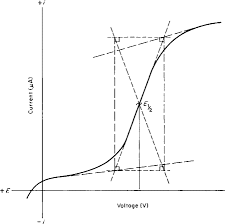To compute for nernst equation, four essential parameters are needed and these parameters are Electrochemical Cell Potential (ΔV°), Number of Electrons (n), Molar Concentration [M1n+] and Molar Concentration [M2n+].

The formula for calculating nernst equation:

ΔV = ΔV° – 0.0592/n log(M1n+/M2n+)

Where:

ΔV = Nernst Equation | Potential
ΔV° = Electrochemical Cell Potential
n = Number of Electrons
M1n+ = Molar Concentration
M2n+ = Molar Concentration

Given an example;
Find the nernst equation when the electrochemical cell potential is 3, the number of electrons is 9, the molar concentration is 6 and the molar concentration is 2.

This implies that;

ΔV° = Electrochemical Cell Potential = 3
n = Number of Electrons = 9
M1n+ = Molar Concentration = 6
M2n+ = Molar Concentration = 2

ΔV = ΔV° – 0.0592/n log(M1n+/M2n+)
ΔV = 3 – (0.0592/9) log(6/2)
ΔV = 3 – (0.00657) log (3)
ΔV = 3 – 0.0065 (0.477)
ΔV = 3 – (0.0031)
ΔV = 2.99

Therefore, the nernst equation is 2.99 V.

Nickzom Calculator – The Calculator Encyclopedia is capable of calculating the nernst equation.

To get the answer and workings of the nernst equation using the Nickzom Calculator – The Calculator Encyclopedia. First, you need to obtain the app.

You can get this app via any of these means:

You can also try the demo version via https://www.nickzom.org/calculator

Apple (Paid) – https://itunes.apple.com/us/app/nickzom-calculator/id1331162702?mt=8
Once, you have obtained the calculator encyclopedia app, proceed to the Calculator Map, then click on Materials and Metallurgical under Engineering.Now, Click on Corrosion under Materials and MetallurgicalNow, Click on Nernst Equation under Corrosion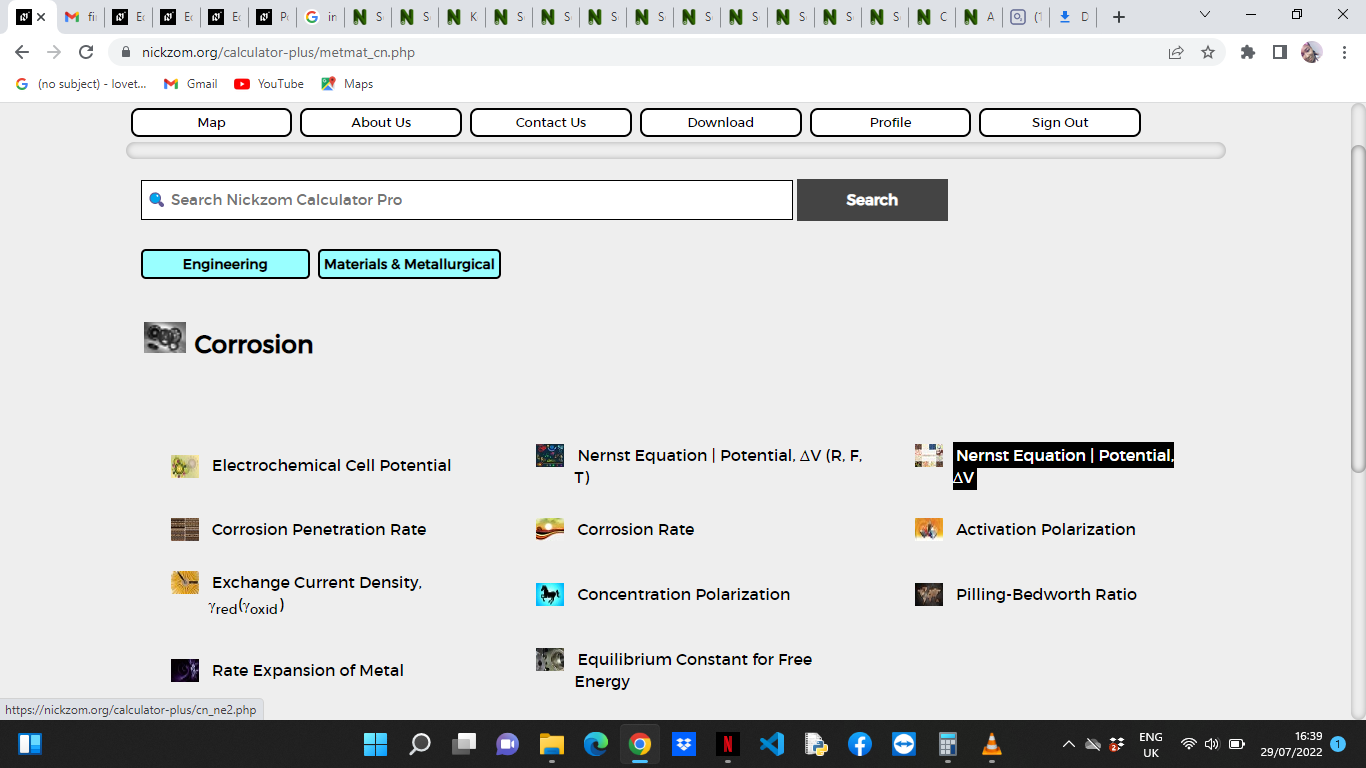The screenshot below displays the page or activity to enter your values, to get the answer for the nernst equation according to the respective parameter which is the Electrochemical Cell Potential (ΔV°), Number of Electrons (n), Molar Concentration [M1n+] and Molar Concentration [M2n+].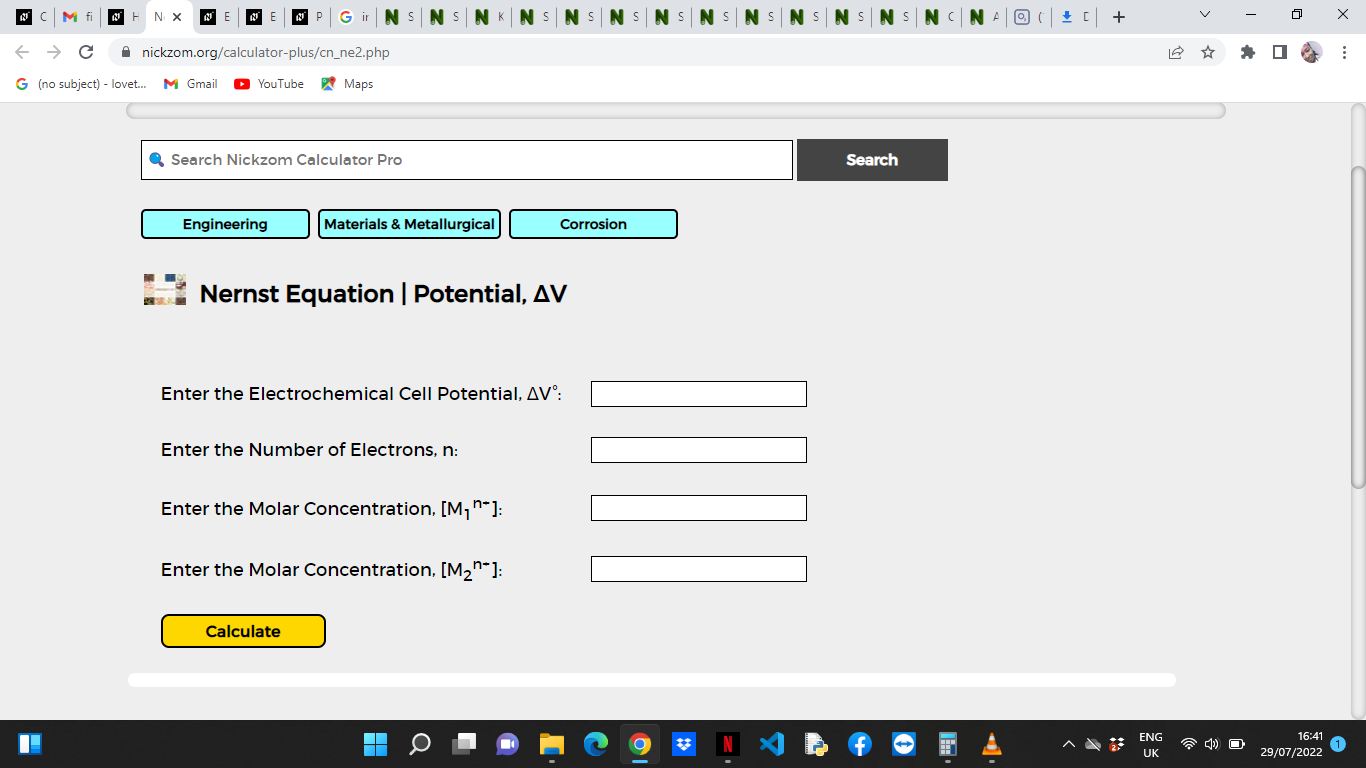Now, enter the values appropriately and accordingly for the parameters as required by the Electrochemical Cell Potential (ΔV°) is 3, Number of Electrons (n) is 9, Molar Concentration [M1n+is 6 and Molar Concentration [M2n+] is 2.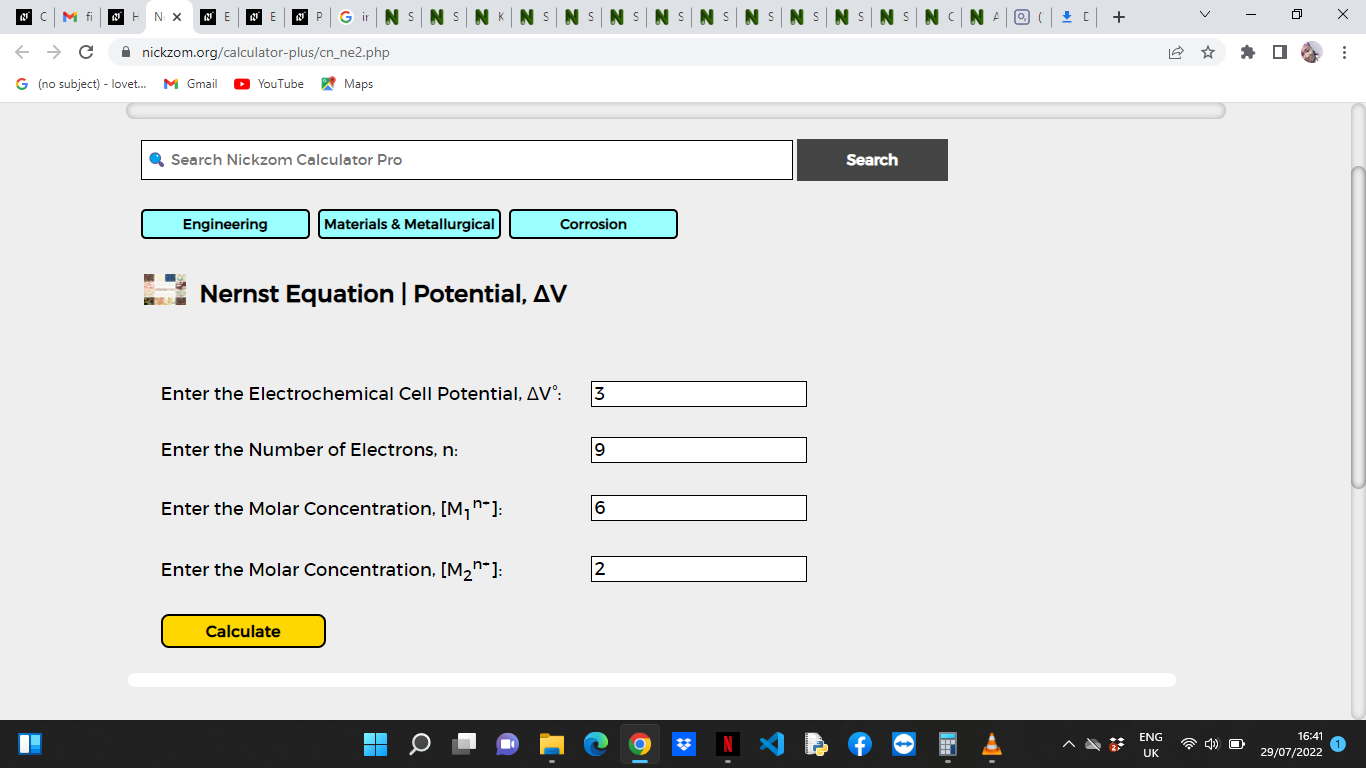Finally, Click on Calculate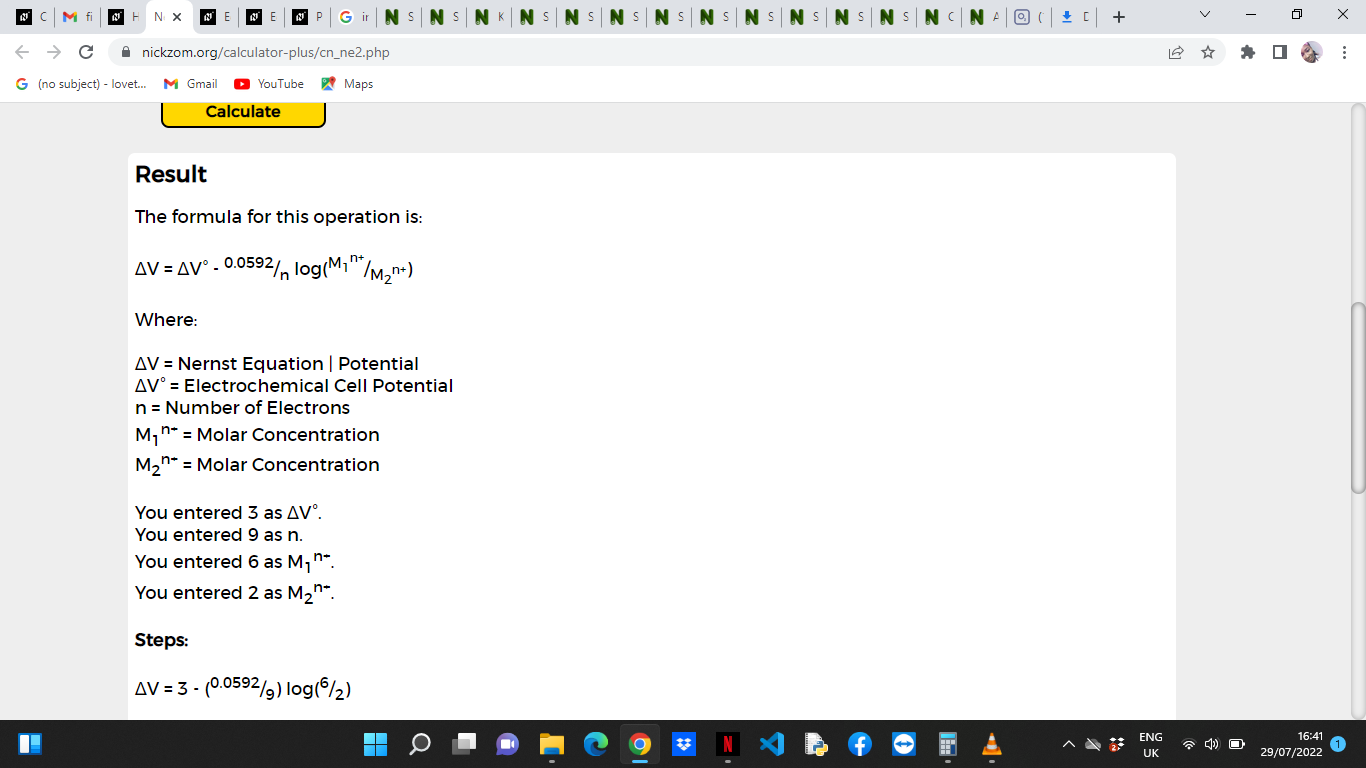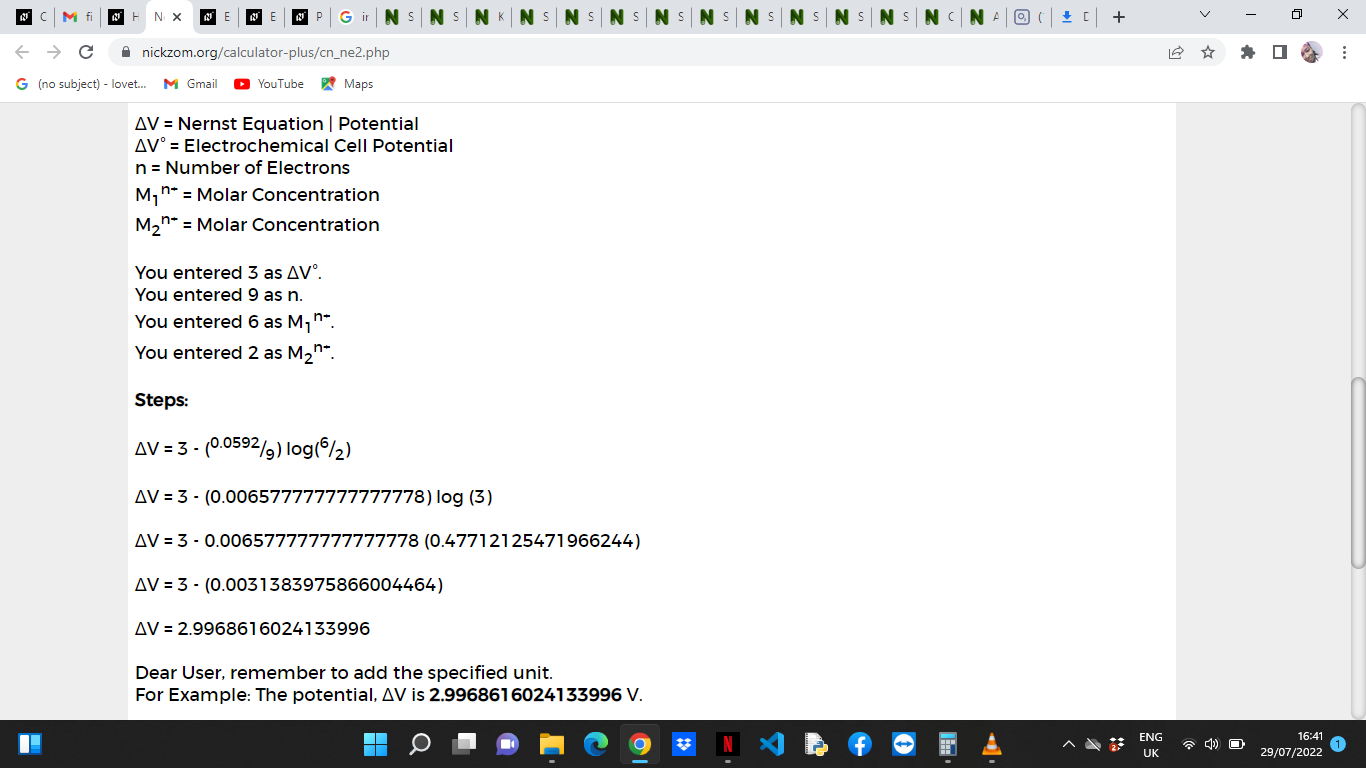As you can see from the screenshot above, Nickzom Calculator– The Calculator Encyclopedia solves for the nernst equation and presents the formula, workings and steps too.